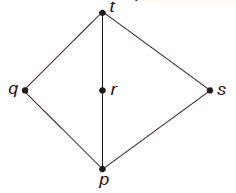# GATE | GATE-CS-2015 (Set 1) | Question 44

Suppose L = {p, q, r, s, t} is a lattice represented by the following Hasse diagram:For any x, y ∈ L, not necessarily distinct, x ∨ y and x ∧ y are join and meet of x, y respectively. Let L3 = {(x,y,z): x, y, z ∈ L} be the set of all ordered triplets of the elements of L. Let pr be the probability that an element (x,y,z) ∈ L3 chosen equiprobably satisfies x ∨ (y ∧ z) = (x ∨ y) ∧ (x ∨ z). Then
(A) Pr = 0
(B) Pr = 1
(C) 0 < Pr ≤ 1/5
(D) 1/5 < Pr < 1

Explanation:

```Number of triplets in L3 = Number of ways in which
we can choose 3 elements
from 5 with repetition

= 5 * 5 * 5
= 125.

Now, when we take x = t, then the given condition for L is
satisfied for any y and z. Here, y and z can be taken in
5 * 5 = 25 ways.

Take x = r, y = p, z = p. Here also, the given condition is
satisfied. So, pr > 25 / 125 > 1/5.

For x = q, y = r, z = s, the given condition is not satisfied
as q ⋁ (r ⋀ s) = q ⋁ p = q, while (q ⋁ r) ⋀ (q ⋁ s) = t ⋀ t = t.

So, pr ≠ 1.

Hence  D choice.
```
My Personal Notes arrow_drop_up
Article Tags :

Be the First to upvote.

Please write to us at contribute@geeksforgeeks.org to report any issue with the above content.